• 字符串合并与拆分 2.1 多列字符串合并 2.2 一列 列表形式的文本合并为一列 2.3 一列字符串与自身合并成为一列 2.4 一列字符串拆分为多列 2.4.1 partition函数 2.4.2 split函数 2.4.3 rsplit函数 3.字符串统计 3.1 ...

1.英文字母大小写转换及填充

s = pd.Series(['lower', 'CAPITALS', 'this is a sentence', 'SwApCaSe'])

• 大写转小写： s.str.lower()
• 小写转大写：s.str.upper()
• 转为新闻标题形式：s.str.title()
• 首字母大写，其余小写：s.str.capitalize()
• 将原来的大写和小写，分别转为小写和大写，即大小写互换：s.str.swapcase()
• 将文字内容用某种字符填充到固定长度，会从两边进行填充：s.str.center(4,’*’)
• 将文字内容用某种字符填充到固定长度，会从文字的右方进行填充，即原来的字符串在左边：s.str.ljust(4,’-’)
• 将文字内容用某种字符填充到固定长度，会从文字的左方进行填充，即原来的字符串在右边：s.str.rjust(4,’-’)
• 在字符串前添加0到指定长度：
s = pd.Series([’-1’, ‘1’, ‘1000’, 10, np.nan])
s.str.zfill(3)

2.字符串合并与拆分

2.1 多列字符串合并

注意：多列字符串在合并时，推荐使用cat函数，该函数是按照索引进行合并的。

s=pd.DataFrame({'col1':['a', 'b', np.nan, 'd'],'col2':['A', 'B', 'C', 'D']})
# 1.有一个缺失值的行不进行合并
s['col1'].str.cat([s['col2']])
# 2.用固定字符(*)替换缺失值，并进行合并
s['col1'].str.cat([s['col2']],na_rep='*')
# 3.用固定字符(*)替换缺失值，并用分隔符(,)进行合并
s['col1'].str.cat([s['col2']],na_rep='*',sep=',')
# 4.索引不一致的合并
#创建series
s = pd.Series(['a', 'b', np.nan, 'd'])
t = pd.Series(['d', 'a', 'e', 'c'], index=[3, 0, 4, 2])
#合并
s.str.cat(t, join='left', na_rep='-')
s.str.cat(t, join='right', na_rep='-')
s.str.cat(t, join='outer', na_rep='-')
s.str.cat(t, join='inner', na_rep='-')

2.2 一列 列表形式的文本合并为一列
s = pd.Series([['lion', 'elephant', 'zebra'], [1.1, 2.2, 3.3], [
'cat', np.nan, 'dog'], ['cow', 4.5, 'goat'], ['duck', ['swan', 'fish'], 'guppy']])
#以下划线进行拼接
s.str.join('_')

使用前：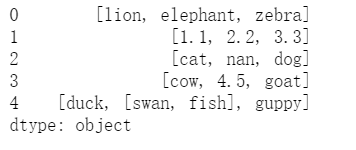使用后：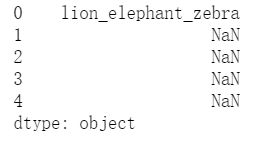2.3 一列字符串与自身合并成为一列
s = pd.Series(['a', 'b', 'c'])
#指定数字
s.str.repeat(repeats=2)
#指定列表
s.str.repeat(repeats=[1, 2, 3])

使用该函数后，效果图分别如下：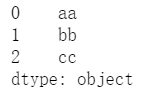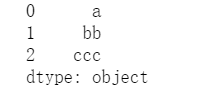2.4 一列字符串拆分为多列
2.4.1 partition函数

partition函数，会将某列字符串拆分为3列，其中2列为值，1列为分隔符。
有两个参数进行设置，分别为：sep(分隔符，默认为空格)，expand(是否生成dataframe，默认为True)

s = pd.Series(['Linda van der Berg', 'George Pitt-Rivers'])
#默认写法,以空格分割，会以第一个分隔符进行拆分
s.str.partition()
#另一写法，会以最后一个分隔符进行拆分
s.str.rpartition()
#以固定符号作为分隔符
s.str.partition('-', expand=False)
#拆分索引
idx = pd.Index(['X 123', 'Y 999'])
idx.str.partition()

2.4.2 split函数

split函数会按照分隔符拆分为多个值。
参数：
pat(分隔符，默认为空格);
n(限制分隔的输出，即查找几个分隔符，默认-1，表示全部);
expend(是否生成dataframe，默认为False)。

s = pd.Series(["this is a regular sentence","https://docs.python.org/3/tutorial/index.html",np.nan])
#1.默认按照空格进行拆分
s.str.split()
#2.按照空格进行拆分，并限制2个分隔符的输出
s.str.split(n=2)
#3.以指定符号拆分，并生成新的dataframe
s.str.split(pat = "/",expand=True)
#4.使用正则表达式来进行拆分，并生成新的dataframe
s = pd.Series(["1+1=2"])
s.str.split(r"\+|=", expand=True)

2.4.3 rsplit函数

如果不设置n的值，rsplit和split效果是相同的。区别是，split是从开始进行限制，rsplit是从末尾进行限制。

s = pd.Series(["this is a regular sentence","https://docs.python.org/3/tutorial/index.html",np.nan])
#区别于split
s.str.rsplit(n=2)

3.字符串统计

3.1 统计某列字符串中包含某个字符串的个数
#1.普通字符
s = pd.Series(['A', 'B','Baca', np.nan])
s.str.count('a')
#2.特殊字符
s = pd.Series(['$', 'B', 'Aab$', 'ca', 'C$B$'])
s.str.count('\$') #3.在索引中进行统计 s=pd.Index(['A', 'A', 'Aaba', 'cat']) s.str.count('a') 3.2 统计字符串长度 s = pd.Series(['dog', '', 5,{'foo' : 'bar'},[2, 3, 5, 7],('one', 'two', 'three')]) s.str.len() 效果图如下：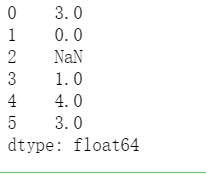4.字符串内容查找(包含正则) 4.1 extract 可通过正则表达式来提取指定内容，小括号内的会生成一列 s = pd.Series(['a1', 'b2', 'c3']) #按照小括号内的进行提取，生成两列 s.str.extract(r'([ab])(\d)') #加上问号后，如果有一个匹配不上，还可以继续匹配 s.str.extract(r'([ab])?(\d)') #可以对生成的新列进行重命名 s.str.extract(r'(?P<letter>[ab])(?P<digit>\d)') #生成1列 s.str.extract(r'[ab](\d)', expand=True) 4.2 extractall 区别于extract，该函数可以提取所有符合条件的元素 s = pd.Series(["a1a2", "b1", "c1"], index=["A", "B", "C"]) #提取所有符合条件的数字,结果为多重索引1列 s.str.extractall(r"[ab](\d)") #提取符合条件的数字，并重命名，结果为多重索引1列 s.str.extractall(r"[ab](?P<digit>\d)") #提取符合条件的a、b和数字，结果为多重索引多列 s.str.extractall(r"(?P<letter>[ab])(?P<digit>\d)") #提取符合条件的a、b和数字，添加问号后，一个匹配不上可以继续向后匹配，结果为多重索引多列 s.str.extractall(r"(?P<letter>[ab])?(?P<digit>\d)") 4.3 find 查询固定字符串在目标字符串中的最小索引。 若需要查询的字符串未出现在目标字符串中，则显示为-1 s = pd.Series(['appoint', 'price', 'sleep','amount']) s.str.find('p') 显示结果如下: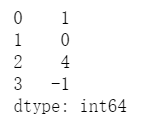4.4 rfind 查询固定字符串在目标字符串中的最大索引。 若需要查询的字符串未出现在目标字符串中，则显示为-1。 s = pd.Series(['appoint', 'price', 'sleep','amount']) s.str.rfind('p',start=1) 查询结果如下：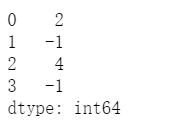4.5 findall 查找系列/索引中所有出现的模式或正则表达式 s = pd.Series(['appoint', 'price', 'sleep','amount']) s.str.findall(r'[ac]') 显示结果如下：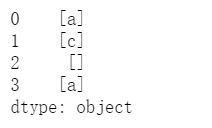4.6 get 从列表、元组或字符串中的每个元素中提取元素的系列/索引。 s = pd.Series(["String", (1, 2, 3), ["a", "b", "c"], 123, -456, {1: "Hello", "2": "World"}]) s.str.get(1) 效果如下图：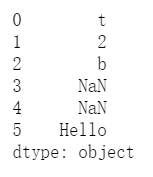4.7 match 确定每个字符串是否与参数中的正则表达式匹配。 s = pd.Series(['appoint', 'price', 'sleep','amount']) s.str.match('^[ap].*t') 匹配效果图如下：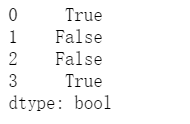5.字符串逻辑判断 5.1 contains函数 测试模式或正则表达式是否包含在系列或索引的字符串中。 参数： pat，字符串或正则表达式； case，是否区分大小写，默认为True,即区分大小写； flags，是否传递到re模块，默认为0; na，对缺失值的处理方法，默认为nan; regex，是否将pat参数当作正则表达式来处理，默认为True。 s = pd.Series(['APpoint', 'Price', 'cap','approve',123]) s.str.contains('ap',case=True,na=False,regex=False) 效果图如下：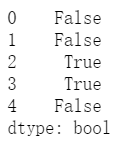5.2 endswith函数 测试每个字符串元素的结尾是否与字符串匹配。 s = pd.Series(['APpoint', 'Price', 'cap','approve',123]) s.str.endswith('e') 匹配结果如下：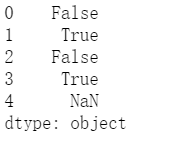处理nan值 s = pd.Series(['APpoint', 'Price', 'cap','approve',123]) s.str.endswith('e',na=False) 效果如下：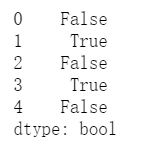5.3 startswith函数 测试每个字符串元素的开头是否与字符串匹配。 s = pd.Series(['APpoint', 'Price', 'cap','approve',123]) s.str.startswith('a',na=False) 匹配如下: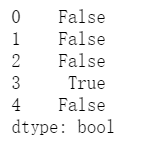5.4 isalnum函数 检查每个字符串中的所有字符是否都是字母数字。 s1 = pd.Series(['one', 'one1', '1', '']) s1.str.isalnum() 效果如下：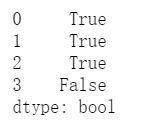5.5 isalpha函数 检查每个字符串中的所有字符是否都是字母。 s1 = pd.Series(['one', 'one1', '1', '']) s1.str.isalpha() 效果如下：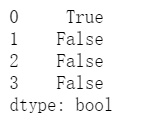5.6 isdecimal函数 检查每个字符串中的所有字符是否都是十进制的。 s1 = pd.Series(['one', 'one1', '1','']) s1.str.isdecimal() 效果如下：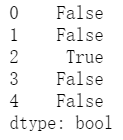5.7 isdigit函数 检查每个字符串中的所有字符是否都是数字。 s1 = pd.Series(['one', 'one1', '1','']) s1.str.isdigit() 效果如下：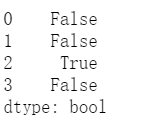5.8 islower函数 检查每个字符串中的所有字符是否都是小写。 s1 = pd.Series(['one', 'one1', '1','']) s1.str.islower() 效果如下：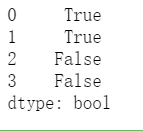5.9 isnumeric函数 检查每个字符串中的所有字符是否都是数字。 s1 = pd.Series(['one', 'one1', '1','','3.6']) s1.str.isnumeric() 效果如下：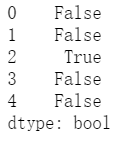5.10 isspace函数 检查每个字符串中的所有字符是否都是空格。 s1 = pd.Series([' one', '\t\r\n','1', '',' ']) s1.str.isspace() 效果如下：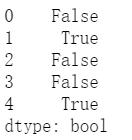5.11 istitle函数 检查每个字符串中的所有字符是否都是标题形式的大小写。 s1 = pd.Series(['leopard', 'Golden Eagle', 'SNAKE', '']) s1.str.istitle() 效果如下：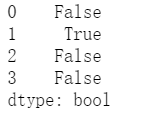5.12 isupper函数 检查每个字符串中的所有字符是否都是大写。 s1 = pd.Series(['leopard', 'Golden Eagle', 'SNAKE', '']) s1.str.isupper() 效果如下：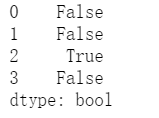5.13 get_dummies函数 按 sep 拆分系列中的每个字符串并返回一个 虚拟/指标变量的dataframe。 s1 = pd.Series(['leopard', 'Golden Eagle', 'SNAKE', '']) s1.str.get_dummies() 效果如下：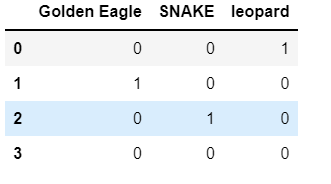该函数还可以进行此类匹配，注意输入的形式 s1=pd.Series(['a|b', np.nan, 'a|c']) s1.str.get_dummies() 效果如下：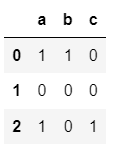6.其他 6.1 strip 删除前导和尾随字符。 s1 = pd.Series(['1. Ant. ', '2. Bee!\n', '3. Cat?\t', np.nan]) s1.str.strip() 效果如下：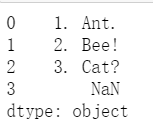6.2 lstrip 删除系列/索引中的前导字符。 6.3 rstrip 删除系列/索引中的尾随字符。 展开全文python 数据分析 pandas 文本处理 • 文本匹配模型汇总 1 DSSM https://posenhuang.github.io/papers/cikm2013_DSSM_fullversion.pdf DSSM是2013年提出来的模型主要应用场景为query与doc的匹配，在这之前，用的更多的还是一些传统的机器学习算法，例如... 文本匹配模型汇总 1 DSSM https://posenhuang.github.io/papers/cikm2013_DSSM_fullversion.pdf DSSM是2013年提出来的模型主要应用场景为query与doc的匹配，在这之前，用的更多的还是一些传统的机器学习算法，例如LSA，BM25等。DSSM也算是深度学习在文本匹配领域中的一个先驱者，接下来我们会先从其结构开始讲起，并简单介绍下其变体。 1.1 模型Term Vector是文本转向量后的值，论文中作者采用的是bag-of-words即词袋模型。然后是Word Hashing层，英文主流文本转vector的方式更多是采用embedding，但是该方法有一个致命的问题就是会出现OOV的问题，而作者提出了一种word hasing的方法，该方法一方面能降低输入数据的维度，其次也能保证不出现OOV的问题，接下来我们详细看下其原理。 1.2 word hashing 这里以good这个单词为例，分为三个步骤 –在good两端添加临界符#good# –采用n-gram的方式分成多个部分，如果是trigrams那么结果是[#go, goo, ood, od#] –最终good将会用[#go, goo, ood, od#]的向量来表示 在英文中，因为只有26个字母，加上临界符是27个符号，3个字母的组合是有限的，即17550种可能，因此也就不会出现OOV的问题。 对于discriminative，discriminate，discrimination 三个单词的意思很像，他们的Word Hashing中也有大部分是相同的。这样两个不同的单词也有可能具有相同的tri-grams，针对这个问题论文中做了统计，这个冲突的概率非常的低，500K个word可以降到30k维，冲突的概率为0.0044%。 可见该方法对于英文来说是有效可行的，但是并不适合中文。 1.3 特征抽取 接下来的结构就很简单了，三个全连接层,激活函数采用的是tanh，把维度降低到128，然后把两个序列的Semantic feature进行了余弦相似度计算，这里给一下前向传播的公式。1.4 输出层 输出层也很简单，一个softmax，因为是计算相似度所以可以看成是二分类，细节不再赘述。 1.5 变体 随着深度学习的发展，CNN、LSTM被提出，这些特征提取的结构也被应用到了DSSM中，其主要的区别就在于把特征提取层的全连接结构换成了CNN或者LSTM。 2 ESIM https://arxiv.org/pdf/1609.06038.pdf ESIM模型主要是用来做文本推理的，给定一个前提premise p 推导出假设hypothesis h，其损失函数的目标是判断 p与 h是否有关联，即是否可以由 p推导出 h，因此，该模型也可以做文本匹配，只是损失函数的目标是两个序列是否是同义句。接下来我们就从模型的结构开始讲解其原理。 2.1 模型 ESIM的论文中，作者提出了两种结构，如下图所示，左边是自然语言理解模型ESIM，右边是基于语法树结构的HIM，本文也主要讲解ESIM的结构，大家如果对HIM感兴趣的话可以阅读原论文。ESIM一共包含四部分，Input Encoding、Local Inference Modeling、 Inference Composition、Prediction，接下来会分别对这四部分进行讲解。 Input Encoding 我们先看一下这一层结构的输入内容，输入一般可以采用预训练好的词向量或者添加embedding层。接下来就是一个双向的LSTM，起作用主要在于对输入值做encoding，也可以理解为在做特征提取，最后把其隐藏状态的值保留下来。 其中i与j分别表示的是不同的时刻，a与b表示的是上文提到的p与h。2.2 Local Inference Modeling 这一层的任务主要是把上一轮拿到的特征值做差异性计算。这里作者采用了attention机制，其中attention weight的计算方法如下：然后根据attention weight计算出a与b的权重加权后的值，计算方法如下：得到encoding值与加权encoding值之后，下一步是分别对这两个值做差异性计算，作者认为这样的操作有助于模型效果的提升，论文有有两种计算方法，分别是对位相减与对位相乘，最后把encoding两个状态的值与相减、相乘的值拼接起来。2.3 Inference Composition 在这一层中，把之前的值再一次送到了BiLSTM中，这里的BiLSTM的作用和之前的并不一样，这里主要是用于捕获局部推理信息 m a m_a m b m_b 及其上下文，以便进行推理组合。 最后把BiLSTM得到的值进行池化操作，分别是最大池化与平均池化，并把池化之后的值再一次的拼接起来。2.4 Prediction 最后把 V送入到全连接层，激活函数采用的是tanh，得到的结果送到softmax层。 2.5 小结 ESIM与BiMPM在相似度匹配任务中都是使用较多的模型，但是ESIM训练速度快，效果也并没有逊色太多，如果加入了语法树，其最终效果也能进一步的提升。 3 ABCNN https://arxiv.org/pdf/1512.05193.pdf 以CNN与attention机制做文本匹配的模型即ABCNN。 在文本任务上，大部分模型均是采用以LSTM为主的结构，本文的作者采用了CNN的结构来提取特征，并用attention机制进行进一步的特征处理，作者一共提出了三种attention的建模方法，下文会详细介绍。 在开始讲解之前，我们简单说明下attention机制，例如我们有两个序列A与B，当我们需要进行相似度比较时，A序列某个时刻的值该和B序列哪个时刻比较最合适呢，而attention机制就是为了解决这个问题的，把B序列的每个时刻的值做了一个加权平均，用加权平均之后的值与A进行比较，说白了attention就是一个加权平均的过程，其中的权重就是BP过程中不断更新得到的。 论文中，作者分为了两个部分进行介绍，首先是没有attention仅有CNN的基础模型BCNN，后续又介绍了添加了attention的ABCNN，其中又分别介绍了三种模式，下文也会以该顺序进行讲解。 3.1 BCNN BCNN的结构如下图所示可以看到BCNN包括四部分，分别是Input Layer、Convolution Layer、Average Pooling Layer、Output Layer，第一层为Input Layer，第二层与第三层为卷积与池化层，最后是用LR作为Output Layer 3.1.1 Input Layer Input Layer即输入层，该层主要是做词嵌入，论文中作者采用的是word2vec的方法，当然，也可以采用glove等其他方法，或者直接输入one-hot，并添加embedding层。作者设置的词向量维度是300维，图中所示，左边序列是5个词维度为[5,300]，右边序列是7个词维度为[7,300]，注意这里作者输入的维度是不固定的，后续的池化层会将维度处理为一样的。 3.1.2 Convolution Layer Convolution Layer即卷积层，在文本处理中，卷积核的大小通常为[n, embedding_size]，n指的是节点的个数，可以理解为n-gram，从而从字/词特征中提取出短语特征，而这里的卷积层和大家平时使用的卷积还不太一样，这里采用了Wide Convolution宽卷积，我们看个图如果不做padding，边缘位置只被卷积了一次，可能损失信息，因此对边缘进行padding,使得边缘与其他位置卷积的次数相同。 3.1.3 Average Pooling Layer Average Pooling Layer即平均池化层，仔细看，图中的第二层和第三层的池化层是不一样的，第二层的池化层是w-ap，第三层是all-ap，有什么区别呢，w-ap的核大小是可以自己定义的即[n, 300]，，其操作过程类似卷积，只不过是取平均值，而all-ap的核大小是和输入的值的维度相同的，这样也就保证了输出的维度是[1, 300]，从而使两个序列在比较的时候的值的维度是相同的。 3.1.4 Output Layer Output Layer即输出层，很简单的LR做一个二分类，不再赘述。 这就是BCNN的全部内容，接下来我们看加了attention的BCNN即ABCNN 3.6 ABCNN ABCNN作者提出了三种结构，我们分别讲解。 3.6.1 ABCNN-1第一种attention的方法是对representation进行了处理，首先，针对两个不同的序列，生成attention matrix A，如图中所示，这个矩阵可以保存了序列0与序列1每一个词/字向量的相似度，论文中作者采用的是欧氏距离，其计算过程为 1 / ( 1 + ∣ x − y ∣ ) 1/(1 + ∣x−y∣) ,其中 ∣ x − y ∣ ∣x−y∣ 表示的是欧氏距离，还是以图中为例，0序列的长度是5，1序列的长度是7，我们需要计算的是0序列中5个节点分别与1序列的7个节点的相似度的值，所以A最终的维度是[5, 7]，这个相似度的值我个人认为还可以采用余弦相似度或者直接两个矩阵相乘（归一化后的值直接相乘就是余弦相似度，所以两个矩阵直接相乘得到的结果理论上来说也可以表示相似度），不过论文中提到欧式距离效果更好一些。 attention matrix生成好后，就能计算attention feature了，计算方法是定义一个权重矩阵W，如图中所示0序列对应的是 W 0 W_0 ,1序列对应的是 W 1 W_1 ，W的维度为[d, s]，d表示的是词/字向量维度，论文中是300，s表示的是序列长度，然后用这个权重矩阵和attention matrix相乘，所以最终的attention feature为最终得到的attention feature维度和representation feature的维度是相同的。 3.6.2 ABCNN-2第二种attention是对卷积之后的结果进行操作，attention matrix的生成方法和1的一样，生成完成后，会分别针对列与行进行求和，还是以图中为例，卷积0的维度为[7, 300]，卷积1的维度为[9,300]，matrix的维度是[7, 9]，生成的求和权重为[7, 1]与[1, 9]，之后的pooling的操作不是简单的求平均，而是换成了把对应的行和求和的权重进行对位相乘，所以最后池化后的结果维度和输入维度是相同的。 ABCNN1与ABCNN2有三个主要的区别 1是对卷积之前的值进行处理，2是对池化之前的值进行处理 1需要两个权重矩阵 W 0 W_0 W 1 W_1 ，参数多一些，更容易过拟合，2不存在这个问题,由于池化是在卷积之后执行的，因此其处理的粒度单元比卷积大，在卷积阶段，获取到的是词/字向量，而池化层获取到的是短语向量，而这个短语向量的维度主要看卷积层的卷积核大小，因此，1和2其实是在不同的粒度上进行了处理。 ABCNN-3第三种方式就是把第一种和第二种方式结合起来 待更 4 BiMPM 5 DIIN 6 DRCN 7 SiaGRU https://aaai.org/ocs/index.php/AAAI/AAAI16/paper/view/12195/12023 8 RE2 https://www.aclweb.org/anthology/P19-1465.pdf 对话匹配模型 Multi-view先CNN卷每个句子，然后使用LSTM卷每个句子的cnn后的表示过滤噪声，从而用最后一个时刻的隐状态作为表示，与回复经过卷积池化后的表示进行相似度计算，SMN 交互，即与之前不同的是，之前的是将整个对话不论几句，都将所有句子处理完后形成一个表示，然后用这个表示与回复计算相似度，而交互的则是将回复的表示与对话中的每个句子均进行一个交互与Multi-view模型类似, 这里作者也考虑同事提取词汇级和语句级的特征, 分别得到两个匹配矩阵M1和M2, 具体的: • Word-Matching-M1: 对两句话的词做word embedding, 再用dot(ei,ej)计算矩阵元素 • Utterance-Matching-M2: 对两句话的词做word embedding, 再过一层GRU提取隐状态变量h, 然后用dot(hi,A*hj)计算矩阵元素 得到的两个匹配矩阵可视为两个通道, 再传给CNN+Pooling获得一组交互特征{vi}. 交互特征传入第二个GRU层, 得到{h’i} 最后的预测层, 作者设计了三种利用隐变量{h’i}的方式: • last: 只用最后一个h’last传入softmax计算score • linearly combined: 将{h’i}线性加权传给softmax • attention: 利用attention机制计算{h’i}的权重 作者的实验表明采用attention的SMN效果最好, 但训练相对最复杂, last最简单且效果基本优于linearly combined。 DAM 对于context和response语义上的联系更进一步，将 attention 应用于多轮对话，打破之前的 RNN 和 CNN 结构，在多轮上速度快，达到了目前最好效果。其次，本文使用 self-attention 和 cross-attention 来提取 response 和 context 的特征展开全文人工智能 深度学习 算法 • 文本embedding的算法大汇总 千次阅读 2019-08-06 17:37:04 文本embedding的前世今生 文章目录文本embedding的前世今生一、潜在语义分析（Latent Semantic Analysis）1. 简单介绍2. 基本原理2.1 词-文档矩阵（Occurences Matrix）2.2 SVD 分解2.3 流程3. 模型评价3.1 具体应用... 文本embedding的算法大汇总 一、潜在语义分析（Latent Semantic Analysis） 1. 简单介绍 LSA 是 1988 年 S.T.Dumais 等人提出的一种新的信息检索代数模型，是用于知识获取和展示的计算理论和方法，和传统向量空间模型（vector space model）一样使用向量来表示词（terms）和文档（documents），并通过向量间的关系（如cos）来判断词及文档间的关系。不同的是，LSA 把高维的向量空间模型（VSM）表示中的文档映射到低维的潜在语义空间中，并用这种潜在的语义结构来表示词和文本，达到消除词之间的相关性和简化文本向量实现降维的目的。 2. 基本原理 通过对大量的文本集进行统计分析，从中提取出词语的上下文使用含义。技术上通过 SVD 分解等处理，消除了同义词、多义词的影响，提高了后续处理的精度。 2.1 词-文档矩阵（Occurences Matrix） LSA 使用词-文档矩阵来描述一个词语是否在一篇文档中。矩阵的行表示词，列表示文档，每一个元素可为该词在文档中的词频或者该词语的 tf-idf（term frequency–inverse document frequency），矩阵的每一行代表该词语的向量表示，每一列代表该文档的向量表示。 2.2 SVD 分解 假设 X 为m*n的矩阵，SVD 就是将 X 分解成 3 个矩阵的乘积： (1.1) X m , n = U m , k ∗ Σ k , k ∗ V n , k T X_{m,n} = U_{m,k}*\Sigma_{k,k}*V^T_{n,k} \tag{1.1} 不妨设 t i T t^T_i 为每第 i i 个词的向量， d j d_j 为第 j j 个文本的向量分解可看成如下的样子：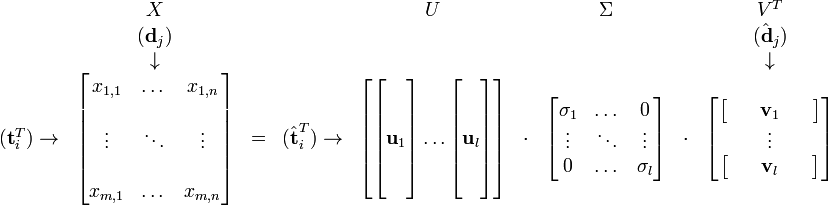其中 σ 1 , . . . , σ l \sigma_1, ... , \sigma_l 被称作奇异值，而 μ 1 , . . . , μ l \mu_1, ... , \mu_l ν 1 , . . . , ν l \nu_1, ... ,\nu_l 则叫做左奇异向量和右奇异向量，可以看出原始矩阵中的 t i t_i 只与 U U 矩阵的第 i i t ^ i \hat t_i 有关， d j d_j 只与 V V 矩阵的第 j j d ^ j \hat d_j 有关，且都由矩阵的所有奇异值所决定。 我们可选取k个最大的奇异值，和它们对应的 U U V V 中的向量相乘，则能得到一个 X X 矩阵的k阶近似，这样就将词向量和文档向量映射到了语义空间，这也是一个从高维空间到低维空间的变换。 2.3 流程 1. 统计分析文档和词的集合，构建词-文档矩阵 A。 2. 对矩阵A做奇异值分解。 3. 对 SVD 分解后得到的矩阵降维。 4. 使用降维后的矩阵构建潜在的语义空间。 3. 模型评价 3.1 具体应用 1. 比较向量 d ^ i \hat d_i d ^ j \hat d_j 可以判断文档 i i 和文档 j j 的相似度，可用于文档聚类和文档分类。 2. 在翻译好的文档上进行训练，可以发现不同语言的相似文档，可用于跨语言检索。 3. 比较向量 t ^ i \hat t_i t ^ j \hat t_j 可以判断词 i i 和词 j j 的相似度，可用于同义词、歧义词检测。 4. 通过查询映射到语义空间，可进行信息检索。给定一个查询字符串，可计算其在语义空间内和已有文档的相关性。 对原始文档，将文档向量映射到语义空间， d ^ j = Σ k − 1 U k T d j \hat d_j = \Sigma^{-1}_k U^T_k d_j ，对查询字符串，得到其对应词的向量后，根据公式 q ^ = Σ k − 1 U k T q \hat q = \Sigma^{-1}_k U^T_k q 将其映射到语义空间，再与文档向量进行比较。 5. 从语义的角度发现词语的相关性，可用于选择题回答模型（multi choice questions answering model） 3.2 优点 1. 低维语义空间可以刻画同义词，同义词会对应着相同或相似的主题。 2. 降维可以除去部分噪声的影响，增加特征的鲁棒性。 3. 充分利用了冗余的数据。 4. 无监督/完全自动化。 5. 与语言无关。 3.3 缺点 1. 新生成的矩阵难以解释。 2. LSA 可以处理向量空间模型无法解决的一义多词（synonymy）问题，但不能解决一词多（polysemy）问题。因为 LSA 将每一个词映射为潜在语义空间中的一个点，也就是说一个词的多个意思在空间中对于的是同一个点，并没有被区分。 3. LSA 的概率模型假设文档和词的分布是服从联合正态分布的，但从观测数据来看是服从泊松分布的。因此 LSA 算法的一个改进 PLSA 使用了多项分布，其效果要好于 LSA。 4. LSA 具有 Bag-of-words model 的缺点，即在一篇文档或者一个句子中忽略词语的先后顺序。 5. SVD 的计算复杂度很高，并且当有新的文档到来时，需重新训练更新模型。 二、神经网络语言模型 1. 简单介绍 用神经网络来训练语言模型的思想最早由百度 IDL （深度学习研究院）的徐伟提出，NNLM（Nerual Network Language Model）是这方面的一个经典模型，具体内容可参考 Bengio 2003年发表在 JMLR上的论文。原文地址：http://jmlr.org/papers/volume3/bengio03a/bengio03a.pdf 相对于传统的语言模型，NNLM 模型使用了低维紧凑的词向量对上文进行表示，这解决了词袋模型带来的数据稀疏、语义鸿沟等问题。显然 NNLM 是一种更好的 n 元语言模型，另一方面在相似的上下文语境中，NNLM 模型可以预测出相似的目标词，而传统模型无法做到这一点。 NNLM 模型直接通过一个神经网络结构对 n 元条件概率进行评估，其基本结构如下：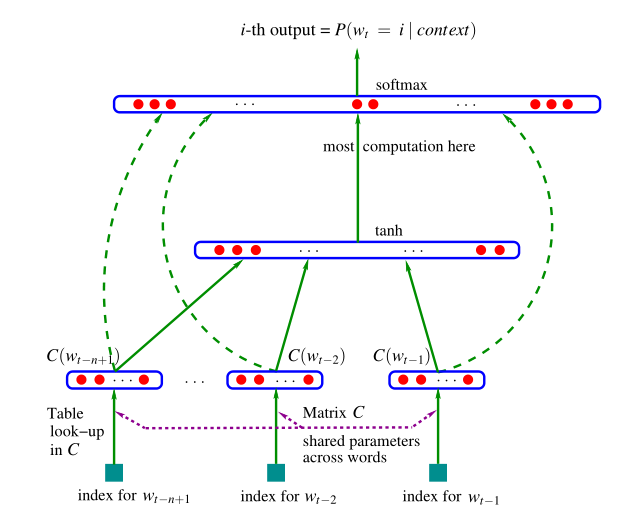2. 基本原理 NNLM 的概率函数是： (2.1) f ( w t , w t − 1 , . . . , w t − n + 2 , w t − n + 1 ) = p ( w t ∣ w 1 t − 1 ) f(w_t,w_{t-1},...,w_{t-n+2},w_{t-n+1})=p(w_t|w^{t-1}_1) \tag{2.1} 给定一段序列时，由其前面的 n-1个词预测第 n 个词的概率。其中 w t w_t 表示第 t 个词， w 1 t − 1 w_1^{t-1} 表示从第一个词到第 t 个词组成的序列，且模型满足： (2.2) { f ( w t , w t − 1 , . . . , w t − n + 2 , w t − n + 1 ) &gt; 0 ∑ i = 1 V f ( w i , w t − 1 , . . . , w t − n + 2 , w t − n + 1 ) = 1 \begin{cases} f(w_t,w_{t-1},...,w_{t-n+2},w_{t-n+1})&gt;0 \\ \sum^V_{i=1}f(w_i,w_{t-1},...,w_{t-n+2},w_{t-n+1})=1 \end{cases} \tag{2.2} 其中 V 为词汇表的大小，即需要满足通过网络预测的每个词的概率都大于0，且所有词的概率之和为1 3. 算法流程 输入：一系列长度为 n 的文本序列训练集，词向量的维度 M，学习率 η \eta 输出：每一个词的词向量 x w x_w • 第一步对训练集进行分词得到词汇表，每一个单词对应一个索引 i i • 第二步随机初始化所有模型参数和映射矩阵 C ∈ R V ∗ N C\in R^{V*N} • 第三步特征映射，通过映射矩阵 C ∈ R V ∗ M C\in R^{V*M} 将每一个词映射成一个特征向量， C ( w i ) ∈ R M C(w_i)\in R^M 表示第 i i 个词的词向量，然后将得到的词向量拼接成一个 ( n − 1 ) M (n-1)M 维的向量（这里我们定义为 h h ）： ( C ( w t − n + 1 ) , . . . , C ( w t − 1 ) ) : = h (C(w_{t-n+1}),...,C(w_{t-1})):=h • 第四步计算条件分布概率：通过一个函数 g g 将输入的词向量序列 h h 转化成一个概率分布 y ∈ R V y\in R^V ，其中第 i i 个元素表示预测的词是第 i i 个词的概率 (2.3) f ( w i , w t − 1 , . . . , w t − n + 2 , w t − n + 1 ) = g ( w i , h ) f(w_i,w_{t-1},...,w_{t-n+2},w_{t-n+1})=g(w_i,h) \tag{2.3} • 第五步定义神经网络输出层输出： (2.4) p ( w t ∣ w t − 1 , . . . , w t − n + 2 , w t − n + 1 ) = e x p ( y w t ) ∑ i V e x p ( y w i ) p(w_t|w_{t-1},...,w_{t-n+2},w_{t-n+1})=\frac{exp(y_{w_t})}{\sum^V_iexp(y_{w_i})} \tag{2.4} 其中 y = b + W + U t a n h ( d + H x ) y=b+W+Utanh(d+Hx) ，模型的参数 θ = ( b , d , W , U , H , C ) , x = h \theta=(b,d,W,U,H,C),x=h 是神经网络的输入。 W ∈ R V ∗ ( n − 1 ) M , H ∈ R Q ∗ ( n − 1 ) M , U ∈ R V ∗ Q W\in R^{V*(n-1)M},H\in R^{Q*(n-1)M},U\in R^{V*Q} ，其中 W W 是可选参数， H H 是输入层到隐藏层的权重矩阵， U U 是隐藏层到输出层的权重矩阵， d , b d,b 是偏置。 • 第六步定义似然函数并更新参数： (2.5) L = 1 T ∑ t l o g f ( w t , w t − 1 , . . . , w t − n + 1 ; θ ) + R ( θ ) L=\frac 1T\sum_tlogf(w_t,w_{t-1},...,w_{t-n+1};\theta)+R(\theta) \tag{2.5} (2.6) θ ← θ + η ∂ l o g p ( w t ∣ w t − 1 , . . . , w t − n + 1 ) ∂ θ \theta \leftarrow\theta + \eta\frac{\partial logp(w_t|w_{t-1},...,w_{t-n+1})}{\partial \theta} \tag{2.6} 其中 R ( θ ) R(\theta) 是正则项 三、词向量模型 Word2Vec 1. 简单介绍 word2vec 模型其实就是一个简单的神经网络，输入层是One-Hot Vector，中间隐藏层没有激活函数，输出层维度和输入层维度一样，用 softmax 回归。这个模型的产物是隐藏层训练好的参数，对应着每一个词的词向量表示。它本质上是一种单词聚类的方法，是实现单词语义推测、句子情感分析等目的一种手段。但是它的 context 窗口很小，没有使用全局的 cooccur，所以实际上对 cooccur 的利用很少。 模型根据输入和输出的定义可分为 CBOW（Continuous Bag-of-Words）与 Skip-Gram 两种模型。CBOW 的输入是某个词的上下文词的词向量，输出是该词的词向量。Skip-Gram 则是与 CBOW 相反，输入是一个词的词向量，输出是该词对应的上下文词的词向量。CBOW 在只适合在少量数据集中训练，而 Skip-Gram 在大型的语料集中表现更好。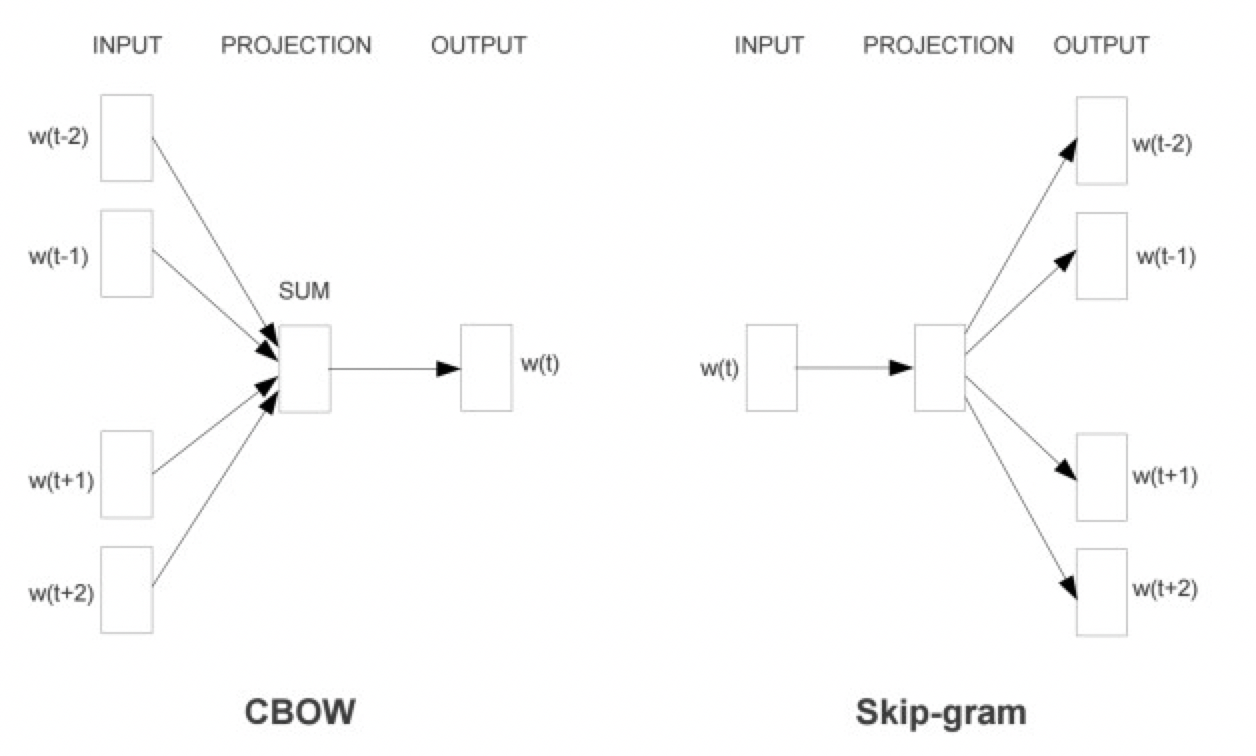2. CBOW 模型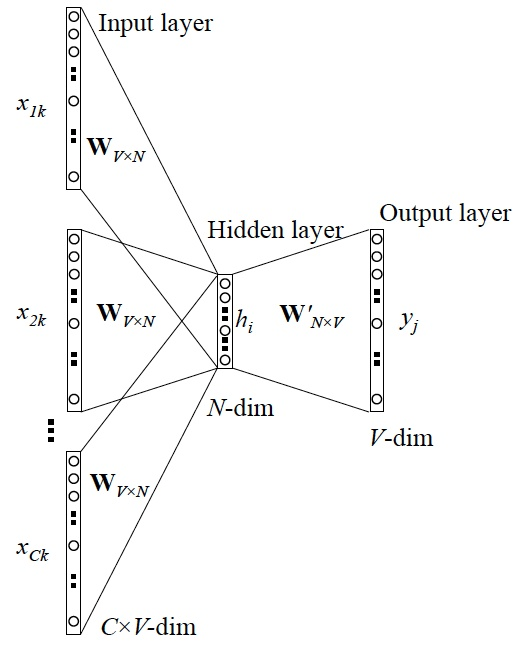输入层是由上下文的词的 One-hot 编码 { x 1 , . . . , x C } \{x_1, ... , x_C\} 组成，其中窗口大小为C，词汇表大小为V，隐藏层是N维的向量，输出是 One-hot 编码的输出单词 y y ，输入的 One-hot 向量通过一个 V × N 维的权重矩阵 W W 连接到隐藏层，再通过一个 N × V 的矩阵 W T W^T 连接到输出层。 2.1 总体算法流程 输入：语料训练样本，词向量的维度大小 N N ，CBOW 的上下文窗口大小 C C ，步长 η \eta 输出：所有词的输入词向量 v v 和输出词向量 v ′ v&#x27; ，即权重矩阵 W W W ′ W&#x27; • 第一步随机初始化模型参数 W W W ′ W&#x27; • 第二步计算隐藏层 h h 的输出： (3.2.1) h = 1 C W T ⋅ ( ∑ i = 1 C x i ) = 1 C ( v w 1 + v w 2 + . . . + v w C ) T h = \frac 1C W^T⋅(\sum^C_{i=1}x_i)=\frac 1C(v_{w_1}+v_{w_2}+...+v_{w_C})^T \tag{3.2.1} • 第三步计算输出层的输入： (3.2.2) u = h ⋅ W ′ u=h\cdot W&#x27; \tag{3.2.2} • 第四步计算输出层的输出： (3.2.3) y c , j = p ( w y , j ∣ w 1 , . . . , w c ) = e x p ( u j ) ∑ j ′ = 1 V e x p ( u j ′ ) y_{c,j} = p(w_{y,j}|w_1,...,w_c) = \frac {exp(u_j)}{\sum^V_{j&#x27;=1}exp(u_{j&#x27;})} \tag{3.2.3} 其中 u j u_j 是输出 u u 的第 j j 个数，即对应的第 j j 个词的概率。 • 第五步定义损失函数，即为给定上下文的输出单词的条件概率，取对数形式： (3.2.4) L o s s = − l o g p ( w O ∣ w I ) = − u j o + l o g ∑ j ′ = 1 V e x p ( u j ′ ) Loss = -logp(w_O|w_I) = -u_{j_o} + log\sum^V_{j&#x27;=1}exp(u_{j&#x27;}) \tag{3.2.4} 其中 j o j_o 是真实输出的词的索引值，我们的目标是最小化 L o s s Loss • 第六步对上述 L o s s Loss 求偏导并更新输出层权重矩阵$W’ 与隐藏层权重矩阵 $W W 直到梯度收敛： (3.2.5) ∂ l o s s ∂ u j = y c , j − t j : = e j \frac {\partial loss}{\partial u_j}=y_{c,j} - t_j:=e_j \tag{3.2.5} 其中 t j t_j j = j o j=j_o 时等于1，其他情况为0 。然后可求出输出层权重的梯度： (3.2.6) ∂ l o s s ∂ W i j ′ = ∂ l o s s ∂ u j ⋅ ∂ u j ∂ W i j ′ = e j ⋅ h i \frac {\partial loss}{\partial W&#x27;_{ij}}=\frac {\partial loss}{\partial u_j}\cdot\frac {\partial u_j}{\partial W&#x27;_{ij}}=e_j\cdot h_i \tag{3.2.6} 则输出层权重更新规则如下： (3.2.7) W i j ′ ( n e w ) = W i j ′ ( o l d ) − η ⋅ e j ⋅ h i W&#x27;^{(new)}_{ij}=W&#x27;^{(old)}_{ij}-\eta\cdot e_j\cdot h_i \tag{3.2.7} 或者： (3.2.8) v w j ′ ( n e w ) = v w j ′ ( o l d ) − η ⋅ e j ⋅ h f o r j = 1 , 2 , . . . , V v&#x27;^{(new)}_{w_j}=v&#x27;^{(old)}_{w_j}-\eta\cdot e_j\cdot h\qquad for\ j=1,2,...,V \tag{3.2.8} 学习率 η &gt; 0 ， e j = y c , j − t j ， h j \eta&gt;0，e_j=y_{c,j}-t_j，h_j 是隐藏层的第 j j 个单元， v w j T v^T_{w_j} 是单词 w j w_j 的输出向量 同理 W W 的梯度： (3.2.9) ∂ l o s s ∂ h i = ∑ j = 1 V ∂ l o s s ∂ u j ⋅ ∂ u j ∂ h i = ∑ j = 1 V e j ⋅ W i j ′ : = E H i \frac {\partial loss}{\partial h_i}=\sum^V_{j=1}\frac {\partial loss}{\partial u_j}\cdot\frac {\partial u_j}{\partial h_i}=\sum^V_{j=1}e_j\cdot W&#x27;_{ij}:=EH_i \tag{3.2.9} 又因为 (3.2.10) h i = 1 C ∑ c = 1 C v w c i = 1 C ∑ c = 1 C ∑ k = 1 V x w c k ⋅ W k i h_i = \frac 1C\sum^C_{c=1}v_{w_c}^i=\frac 1C\sum^C_{c=1}\sum^V_{k=1}x_{w_c}^k\cdot W_{ki} \tag{3.2.10} (3.2.11) ∂ l o s s ∂ W k i = ∂ l o s s ∂ h i ∂ h i ∂ W k i = E H i ⋅ 1 C ∑ c = 1 C x w c k \frac {\partial loss}{\partial W_{ki}}=\frac {\partial loss}{\partial h_i}\frac {\partial h_i}{\partial W_{ki}}=EH_i\cdot \frac 1C\sum^C_{c=1}x^k_{w_c} \tag{3.2.11} 其中 x w c k x^k_{w_c} 指的是第c个单词的One-hot向量的第 k k 个元素的值，所以 (3.2.12) ∂ l o s s ∂ W = ∂ l o s s ∂ h ⋅ ∂ h ∂ W = 1 C x w c ⋅ E H T \frac {\partial loss}{\partial W}=\frac {\partial loss}{\partial h}\cdot\frac {\partial h}{\partial W}=\frac 1Cx_{w_c}\cdot EH^T \tag{3.2.12} 这里我们得到的是一个 V ∗ N V*N 维度的矩阵，又因为对每个 x w c x_{w_c} ，都为只有一个元素值为1、其他都为0的One-hot编码，故 ∂ l o s s / ∂ W {\partial loss}/{\partial W} 矩阵中只有 w c w_c 单词的索引行不为0，行值为 1 C E H \frac 1CEH ，所以 W W 的更新公式为： (3.2.13) v w I , c ( n e w ) = v w I , c ( o l d ) − 1 C ⋅ η ⋅ E H T v^{(new)}_{w_{I,c}}=v^{(old)}_{w_{I,c}}-\frac 1C\cdot\eta\cdot EH^T \tag{3.2.13} 其中 v w I , c ( n e w ) v^{(new)}_{w_{I,c}} 是上下文中第c个单词的输入向量（隐藏层权重的对应单词的行向量） 3. Skip-Gram 模型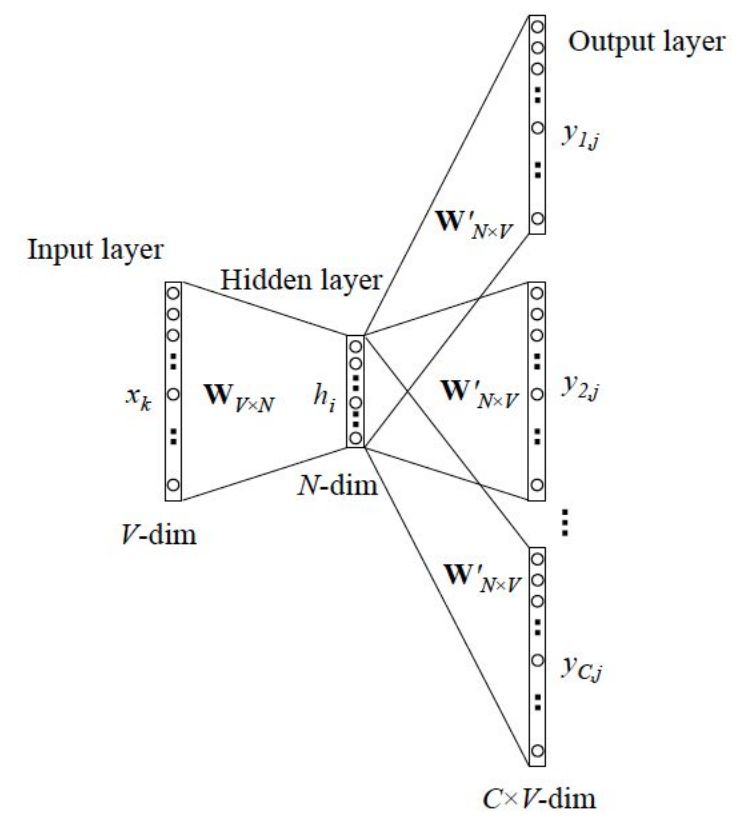Skip-Gram模型是由Mikolov等人提出的。上图展示了Skip-Gram模型的过程，该模型可以看作CBOW模型的逆过程。 3.1 总体算法流程 输入：语料训练样本，词向量的维度大小 N N ，需要预测的上下文窗口大小 C C ，步长 η \eta 输出：所有词的输入词向量 v v 和输出词向量 v ′ v&#x27; ，即权重矩阵 W W W ′ W&#x27; • 第一步随机初始化模型参数 W W W ′ W&#x27; • 第二步计算隐藏层 h h 输出: (3.3.1) h = W ( k , ⋅ ) : = v w I h=W_{(k,\cdot)}:=v_{w_I} \tag{3.3.1} • 第三步计算输出层的输入： (3.3.2) u = h ⋅ W ′ u = h\cdot W&#x27; \tag{3.3.2} • 第四步计算输出层的输出： (3.3.3) y c , j = p ( w c , j = w O , c ∣ w I ) = e x p ( u c , j ) ∑ j ′ = 1 V e x p ( u j ′ ) y_{c,j}=p(w_{c,j}=w_{O,c}|w_I)=\frac {exp(u_{c,j})}{\sum^V_{j&#x27;=1}exp(u_{j&#x27;})} \tag{3.3.3} 这里 w c , j w_{c,j} 是第 c c 个输出， w O , c w_{O,c} 是中心词对应的目标单词中的第 c c 个单词， w I w_I 是中心词（即输入词）， y c , j y_{c,j} 是第 c c 个输出向量的第 j j 个单元的输出值， u c , j u_{c,j} 是第 c c 个输出向量上的第 j j 个单元的输入。且有： (3.3.4) u c , j = u j = v w j ′ T ⋅ h u_{c,j}=u_j=v&#x27;^T_{w_j}\cdot h\tag{3.3.4} v w j ′ T v&#x27;^T_{w_j} 是词汇表第 j j 个单词的输出向量（ W ′ W&#x27; 的第 j j 列） • 第五步定义损失函数： (3.3.5) L o s s = − ∑ c = 1 C u j c ∗ + C ⋅ l o g ∑ j ′ = 1 V e x p ( u j ′ ) Loss=-\sum^C_{c=1}u_{j^*_c}+C\cdot log\sum^V_{j&#x27;=1}exp(u_{j&#x27;})\tag{3.3.5} 其中 j c ∗ j^*_c 表示第 c c 个真实输出单词的索引值 • 第六步对上述 L o s s Loss 求偏导并更新输出层权重矩阵$W’ 与隐藏层权重矩阵 $W W 直到梯度收敛： (3.3.6) ∂ l o s s ∂ u c , j = y c , j − t c , j : = e c , j \frac {\partial loss}{\partial u_{c,j}}=y_{c,j}-t_{c,j}:=e_{c,j}\tag{3.3.6} 我们可定义一个 V 维的向量 E I = { E I 1 , . . . , E I V } EI = \{EI_1,...,EI_V\} ，该向量是 C 个预测单词的误差总和： (3.3.7) E I j = ∑ c = 1 C e c , j EI_j=\sum^C_{c=1}e_{c,j}\tag{3.3.7} (3.3.8) ∂ l o s s ∂ W i j ′ = ∑ c = 1 C ∂ l o s s ∂ u c , j ⋅ ∂ u c , j ∂ W i j ′ = E I j ⋅ h i \frac {\partial loss}{\partial W&#x27;_{ij}}=\sum^C_{c=1}\frac {\partial loss}{\partial u_{c,j}}\cdot\frac {\partial u_{c,j}}{\partial W&#x27;_{ij}}=EI_j\cdot h_i\tag{3.3.8} 输出层权重矩阵 W ′ W&#x27; 的更新公式： (3.3.9) W i j ′ ( n e w ) = W i j ′ ( o l d ) − η ⋅ E I j ⋅ h i W&#x27;^{(new)}_{ij}=W&#x27;^{(old)}_{ij}-\eta\cdot EI_j\cdot h_i\tag{3.3.9} 或者 (3.3.10) v w j ′ ( n e w ) = v w j ′ ( o l d ) − η ⋅ E I j ⋅ h v&#x27;^{(new)}_{w_j}=v&#x27;^{(old)}_{w_j}-\eta\cdot EI_j\cdot h\tag{3.3.10} 隐藏层权重矩阵 W W 的更新公式： (3.3.11) v w I ( n e w ) = v w I ( o l d ) − η ⋅ E H T v^{(new)}_{w_I}=v^{(old)}_{w_I}-\eta\cdot EH^T\tag{3.3.11} 其中 E H EH 是一个N维向量 (3.3.12) E H i = ∑ j = 1 V E I j ⋅ W i j ′ EH_i=\sum^V_{j=1}EI_j\cdot W&#x27;_{ij}\tag{3.3.12} 4. 模型的优化方法 对上述模型，每个单词都存在两类向量的表达，即输入向量 v w v_w （输入层到隐藏层的权重矩阵 W W ），输出向量 v w ′ v&#x27;_w （隐藏层到输出层的权重矩阵 W ′ W&#x27; ）。学习得到输入向量比较简单，但是学习输出向量是很困难的，需要遍历词汇表中的每个单词。若词汇表非常巨大，那么计算是非常庞大的。 为了解决计算量太大的问题，我们有两种改进的优化方法：分层 softmax（Hierarchical softmax）和负采样（negative sampling）。 4.1 Hierarchical softmax 为了避免计算词汇表所有词的 softmax 概率，分层 softmax 采用霍夫曼树（huffman）来代替隐藏层到输出 softmax 层的映射。即将上述的输出层权重矩阵 W ′ W&#x27; 替换成 霍夫曼树的隐节点的权重 θ \theta 由于霍夫曼树是二叉树，故计算量由之前的 V 变成 l o g 2 V log_2V ，而且我们仍然有差不多同样的模型参数（原始模型：V 个单词的输出向量，分层 softmax：V - 1 个隐节点的输出向量）。且依据每个单词的词频作为权重构建的霍夫曼树，高频词的路径更短，更容易被找到。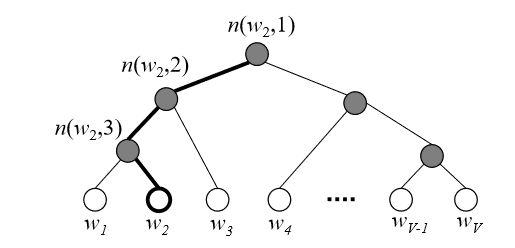这里树的所有内部节点就类似之前的神经网络隐藏层的神经元。根节点的词向量对应我们投影后的词向量，而所有叶子节点就类似之前 softmax 输出层的神经元，叶子节点的个数就是词汇表的大小。这里从隐藏层到输出层的 softmax 映射不是一下就完成的，是沿着霍夫曼树一步一步完成的。每一个隐节点都是一个二分类的逻辑回归问题，往左子树走为负类（霍夫曼编码为1），右边则为正类（编码为0），激活函数用 sigmoid 函数即： (3.4.1) P ( + ) = σ ( x w T θ ) = 1 1 + e x p ( − x w T θ ) P(+)=\sigma(x^T_w\theta)=\frac 1{1+exp(-x^T_w\theta)}\tag{3.4.1} 其中 x w x_w 是当前内部节点的词向量， θ \theta 是我们需要训练得到的模型参数 4.1.1 模型参数的梯度计算 分层 softmax 没有单词的输出向量，而是 V - 1 个隐节点都有一个输出向量 v n ( w , j ) ′ v&#x27;_{n(w,j)} 。首先定义经过霍夫曼树某一个节点 j j 的逻辑回归概率： (3.4.2) P ( d j w ∣ x w , θ j − 1 w ) = { σ ( x w T θ j − 1 w ) d j w = 0 1 − σ ( x w T θ j − 1 w ) d j w = 1 P(d^w_j|x_w,\theta^w_{j-1})= \begin{cases} \sigma(x^T_w\theta^w_{j-1}) &amp; \text d_j^w=0\\ 1-\sigma(x^T_w\theta^w_{j-1}) &amp; \text d_j^w=1 \end{cases}\tag{3.4.2} 那么一个单词作为输出词的最大似然为： (3.4.3) p ( w = w O ) = ∏ j = 2 L ( w ) P ( d j w ∣ x w , θ j − 1 w ) = ∏ j = 2 L ( w ) [ σ ( x w T θ j − 1 w ) ] 1 − d j w [ 1 − σ ( x w T θ j − 1 w ) ] d j w p(w=w_O)=\prod^{L(w)}_{j=2}P(d^w_j|x_w,\theta^w_{j-1}) =\prod^{L(w)}_{j=2}[\sigma(x^T_w\theta^w_{j-1})]^{1-d_j^w}[1-\sigma(x^T_w\theta^w_{j-1})]^{d_j^w}\tag{3.4.3} 取对数： (3.4.4) L = l o g ∏ j = 2 L ( w ) P ( d j w ∣ x w , θ j − 1 w ) = ∑ j = 2 L ( w ) ( ( 1 − d j w ) l o g [ σ ( x w T θ j − 1 w ) ] + d j w l o g [ 1 − σ ( x w T θ j − 1 w ) ] ) L=log\prod^{L(w)}_{j=2}P(d^w_j|x_w,\theta^w_{j-1}) =\sum^{L(w)}_{j=2}((1-d_j^w)log[\sigma(x^T_w\theta^w_{j-1})]+d_j^wlog[1-\sigma(x^T_w\theta^w_{j-1})])\tag{3.4.4} 于是可对模型参数求偏导： (3.4.5) ∂ L ∂ θ j − 1 w = ( 1 − d j w − σ ( x w T θ j − 1 w ) ) x w \frac{\partial L}{\partial \theta^w_{j-1}}=(1-d_j^w-\sigma(x^T_w\theta^w_{j-1}))x_w\tag{3.4.5} 同理 (3.4.6) ∂ L ∂ x w = ( 1 − d j w − σ ( x w T θ j − 1 w ) ) θ j − 1 w \frac{\partial L}{\partial x_w}=(1-d_j^w-\sigma(x^T_w\theta^w_{j-1}))\theta^w_{j-1}\tag{3.4.6} 4.1.2 基于分层 softmax 的 CBOW 模型 假设我们取得上下文的窗口大小为 2 c 2c ，即训练样本中的每一个词都以其前面和后面 c c 个词作为输入，该词本身作为样本输出。 算法流程如下： 输入：基于 CBOW 的语料训练样本，词向量维度的大小 N N ，CBOW 的上下文大小 2 c 2c ，步长 η \eta 输出：huffman 树的所有内部节点模型参数 θ \theta 和所有的词向量 x x • 第一步基于语料库构建霍夫曼树树 • 第二步随机初始化模型参数 θ \theta 和所有词的词向量 x x • 第三步计算梯度并对每个训练集中的样本 ( c o n t e x t ( w ) , w ) (context(w),w) 作如下处理： 1. e = 0 e=0 ，计算 KaTeX parse error: Can't use function '$' in math mode at position 50: …\tag{3.4.7} 其中 $̲x_i$ 为上下文第 $i$ …

其中 x i x_i 为上下文第 i i 个词的输入词向量

2. f o r   j = 2   t o   L ( w ) for\ j=2\ to\ L(w) 计算：
f = σ ( x w T ) θ j − 1 w g = ( 1 − d j w − f ) η e = e + g θ j − 1 w θ j − 1 w = θ j − 1 w + g x w f=\sigma(x^T_w)\theta^w_{j-1} \\ g=(1-d^w_j-f)\eta \\ e=e+g\theta^w_{j-1} \\ \theta^w_{j-1}=\theta^w_{j-1}+gx_w

3. 对于 c o n t e x t ( w ) context(w) 中的每一个词向量 x i x_i 进行更新直到梯度收敛：
x i = x i + e x_i = x_i+e

4.1.3 基于分层 softmax 的 Skip-Gram 模型

对于 Skip-Gram 模型来说，输入只有一个词 w w ，输出为 2 c 2c 个词向量 c o n t e x t ( w ) context(w) ，我们期望 P ( x i ∣ x w ) , i = 1 , 2 , . . . , 2 c P(x_i|x_w),i=1,2,...,2c 最大。

我们在期望 P ( x i ∣ x w ) , i = 1 , 2 , . . . 2 c P(x_i|x_w),i=1,2,...2c 最大时，也就是期望 P ( x w ∣ x i ) , i = 1 , 2 , . . . , 2 c P(x_w|x_i),i=1,2,...,2c 最大，在训练时，word2vec 使用了后者，因为这样可以在一次迭代时不是只更新 x w x_w 一个词的词向量，而是 x i , i = 1 , 2 , . . . , 2 c x_i,i=1,2,...,2c 2 c 2c 个词的词向量，可以使得整体的迭代更加均衡。所以 Skip-Gram 模型不像 CBOW 模型对输入进行更新，而是对 2 c 2c 个输出进行更新。

这里相当于把每一个原本的输出词向量作为输入，原本的输入词向量作为输出，类似上下文大小为1的 CBOW 模型，依次更新每一个输出的词向量。

算法流程如下：

输入：基于 Skip-Gram 的语料训练样本词向量维度的大小 N N ，Skip-Gram 的上下文大小 2 c 2c ，步长 η \eta

输出：huffman 树的所有内部节点模型参数 θ \theta 和所有的词向量 x x

• 第一步基于语料库构建霍夫曼树

• 第二步随机初始化模型参数 θ \theta 和所有词的词向量 x x

• 第三步对每一个样本 ( w , c o n t e x t ( w ) ) (w,context(w)) 做如下处理：

1. $for\ i=1\ to\ 2c$:

e = 0 , f o r   j = 2   t o   L ( w ) e=0,for\ j=2\ to\ L(w) ，计算：
f = σ ( x i T θ j − 1 w ) g = ( 1 − d j w − f ) η e = e + g θ j − 1 w θ j − 1 w = θ j − 1 w + g x i f=\sigma(x^T_i\theta^w_{j-1}) \\ g=(1-d^w_j-f)\eta \\ e=e+g\theta^w_{j-1} \\ \theta^w_{j-1}=\theta^w_{j-1}+gx_i
更新每个该词的词向量：
x i = x i + e x_i=x_i+e

2. 若梯度收敛则结束，否则回到步骤1继续迭代

这里与上面 CBOW 模型的区别在于，上面 CBOW 其实也是由 2 c 2c 个上下文词向量来走到 Huffman 树的叶子节点，但是他的根节点为 2 c 2c 个词向量的求和均值，并且更新的也是 c o n t e x t ( w ) context(w) 中的 2 c 2c 个词向量。而 Skip-Gram 每次单一的输入 2 c 2c 个词向量中的一个，最后更新的也是这个输入的词向量和Huffman内部节点的参数。

4.2 Negative Sampling

相比于分层 softmax ，负采样没有用到霍夫曼树，而是通过采样得到 neg 个负例加上一个真实的正例，进行二元逻辑回归，得到负采样对应每个词 w i w_i 对应的模型参数 θ i \theta_i ，以及每个词的词向量。负采样每次让一个训练样本仅仅更新一小部分的权重参数，从而降低梯度下降过程中的计算量。

4.2.1 负采样的方法

若词汇表大小为 V，我们先将长度为1的线段分成 V 份，每一份对应一个词，且词频越高对应线段长度越长，词 w w 的长度：
l e n ( w ) = c o u n t ( w ) ∑ u ∈ v o c a b c o u n t ( u ) len(w)=\frac{count(w)}{\sum_{u\in vocab}count(u)}
在word2vec中长度计算如下：
l e n ( w ) = c o u n t ( w ) 3 / 4 ∑ u ∈ v o c a b c o u n t ( u ) 3 / 4 len(w)=\frac{count(w)^{3/4}}{\sum_{u\in vocab}count(u)^{3/4}}
采样前，我们将线段均匀划分成 M（默认为 1 0 8 10^8 ）份，且 M >> V，这样每个划分点 m i , i = 0 , 1 , 2 , . . . , M m_i,i=0,1,2,...,M 都对会落在某一个词的线段上，我们只需要从这 M+1 个点上采样出 neg 个位置就行，其对应的词就是我们需要的负例，且注意不要采到正例。

4.2.2 模型参数的梯度计算

假设通过负采样，我们得到 n e g neg 个负例 ( c o n t e x t ( w ) , w i ) , i = 1 , 2 , . . . , n e g (context(w),w_i),i=1,2,...,neg ，并假设正例词为 w 0 w_0

那么我们正例和负例期望满足：
P ( c o n t e x t ( w 0 ) , w i ) = σ ( x w 0 T θ w i ) , y i = 1 , i = 0 P ( c o n t e x t ( w 0 ) , w i ) = 1 − σ ( x w 0 T θ w i ) , y i = 0 , i = 1 , 2 , . . . , n e g P(context(w_0),w_i)=\sigma(x^T_{w_0}\theta^{w_i}),\quad y_i=1,i=0 \\ P(context(w_0),w_i)=1-\sigma(x^T_{w_0}\theta^{w_i}),\quad y_i=0,i=1,2,...,neg
最大似然为：
P ( w = w 0 ) = ∏ i = 0 n e g P ( c o n t e x t ( w 0 ) , w i ) = ∏ i = 0 n e g [ σ ( x w 0 T θ w i ) ] y i [ 1 − σ ( x w 0 T θ w i ) ] 1 − y i P(w=w_0)=\prod^{neg}_{i=0}P(context(w_0),w_i) =\prod^{neg}_{i=0}[\sigma(x^T_{w_0}\theta^{w_i})]^{y_i}[1-\sigma(x^T_{w_0}\theta^{w_i})]^{1-y_i}
取对数
L = ∑ i = 0 n e g y i l o g ( σ ( x w 0 T θ w i ) ) + ( 1 − y i ) l o g ( 1 − σ ( x w 0 T θ w i ) ) L=\sum^{neg}_{i=0}y_ilog(\sigma(x^T_{w_0}\theta^{w_i}))+(1-y_i)log(1-\sigma(x^T_{w_0}\theta^{w_i}))
首先计算 θ w i \theta^{w_i} 的梯度：
∂ L ∂ θ w i = y i ( 1 − σ ( x w 0 T θ w i ) ) x w 0 − ( 1 − y i ) σ ( x w 0 T θ w i ) x w 0 = ( y i − σ ( x w 0 T θ w i ) ) x w 0 \frac{\partial L}{\partial \theta^{w_i}}=y_i(1-\sigma(x^T_{w_0}\theta^{w_i}))x_{w_0}-(1-y_i)\sigma(x^T_{w_0}\theta^{w_i})x_{w_0} =(y_i-\sigma(x^T_{w_0}\theta^{w_i}))x_{w_0}
同理可得 x w 0 x_{w_0} 的梯度：
∂ L ∂ θ w 0 = ∑ i = 0 n e g ( y i − σ ( x w 0 T θ w i ) ) θ w 0 \frac{\partial L}{\partial \theta^{w_0}}= \sum^{neg}_{i=0}(y_i-\sigma(x^T_{w_0}\theta^{w_i}))\theta^{w_0}

4.2.3 基于负采样的 CBOW 模型

假设我们取得上下文的窗口大小为 2 c 2c ，即训练样本中的每一个词都以其前面和后面 c c 个词作为输入，该词本身作为样本输出。

算法流程如下：

输入：语料训练样本，词向量维度的大小 N N ，CBOW 的上下文窗口大小 2 c 2c ，步长 η \eta ，以及负采样的个数 $neg$

输出：词汇表每个词对应的模型参数 θ \theta 和所有的词向量 x x

• 第一步随机初始化所有的模型参数 θ w \theta^w ，所有的词向量 x w x_w

• 第二步对每个训练样本 c o n t e x t ( w 0 ) , w 0 ) context(w_0),w_0) ，进行负采样，得到 n e g neg 个负例词 $w_i,i=1, 2,…,neg$

• 第三步进行梯度上升迭代过程，对训练语料中的每一个样本 ( c o n t e x t ( w 0 ) , w 0 , w 1 , . . . , w n e g ) (context(w_0),w_0,w_1,...,w_{neg}) 做如下处理:

1. e = 0 e=0 ，计算隐含层输出：
x w 0 = 1 2 c ∑ i = 1 2 c x i x_{w_0}=\frac 1{2c}\sum ^{2c}_{i=1}x_i

2. f o r   i = 0   t o   n e g for\ i=0\ to\ neg ，计算：
f = σ ( x w 0 T θ w i ) g = ( y i − f ) η e = e + g θ w i θ w i = θ w i + g x w 0 f=\sigma(x^T_{w_0}\theta^{w_i}) \\ g=(y_i-f)\eta \\ e = e+g\theta^{w_i} \\ \theta^{w_i}=\theta^{w_i}+gx_{w_0}

3. 根据梯度对 c o n t e x t ( w ) context(w) 中的每一个词向量 x k x_k （2c 个）进行更新：
x k = x k + e x_k = x_k+e

4. 若梯度收敛，结束迭代，否则回到第三步进行迭代更新

4.2.4 基于负采样的 Skip-Gram 模型

与基于层级 softmax 的 Skip-Gram 模型一样，这里也是对 2 c 2c 个输出词向量进行迭代更新。

算法流程如下：

输入：基于 Skip-Gram 的语料训练样本，词向量的维度大小 N，Skip-Gram 的上下文大小 2 c 2c ，步长 η \eta ，负采样的个数 n e g neg

输出：词汇表每个词对应的模型参数 θ w \theta^w ，所有词向量 x w x_w

• 第一步随机初始化所有的模型参数 θ \theta 和词向量 x x

• 第二步对每个训练样本 ( c o n t e x t ( w 0 ) , w 0 ) (context(w_0),w_0) 采样出 n e g neg 个负例词 w i , i = 1 , 2 , . . . , n e g w_i,i=1,2,...,neg

• 第三步进行梯度上升，并更新参数，对每个样本 ( c o n t e x t ( w 0 ) , w 0 , w 1 , . . . , w n e g ) (context(w_0),w_0,w_1,...,w_{neg}) 做如下处理：

1. f o r   i = 1   t o   2 c : for\ i=1\ to\ 2c:

e = 0 ， f o r   j = 0   t o   n e g e=0，for\ j=0\ to\ neg ，计算：
f = σ ( x w 0 T θ w j ) g = ( y j − f ) η e = e + g θ w j θ w j = θ w j + g x w 0 i f=\sigma(x^T_{w_0}\theta^{w_j}) \\ g=(y_j-f)\eta \\ e=e+g\theta^{w_j} \\ \theta^{w_j}=\theta^{w_j}+gx_{w_{0i}} \\
利用梯度对该输出词向量进行更新：
x w 0 i = x w 0 i + e x_{w_0}^i=x_{w_0}^i+e
其中 x w 0 i x^i_{w_0} 为中心词为 w 0 w_0 的上下文 2 c 2c 个词中的第 i i 个词的词向量

2. 若梯度收敛，结束迭代，否则回到1继续迭代更新参数

四、GloVe

1. 简单介绍

GloVe 全称叫 Global Vectors for Word Representation，是一个基于全局词频统计（count-based&overall statistics）的词表征（word representation）工具，与 word2vec 一样，她也是将每一个词表示成一个向量。

GloVe 结合了 LSA 和 word2vec 两者的优点，充分利用了所有语料全局信息，更易于优化且训练速度更快，但仅仅只关注了词语的共现关系，忽略了词语的顺序关系，因此训练出来的词向量包含的语义信息有限，只能进行一些词语相似度等有限的任务。

2. 基本原理

GloVe 的实现可分为三步：

• 根据语料库构建一个共现矩阵（Co-ocurrence Matrix） X X

• 构建词向量和共现矩阵之间的近似关系，论文作者提出的关系式为：
(4.1) w i T w ‾ j + b i + b ‾ j = l o g ( X i j ) w^T_i\overline w_j+b_i+\overline b_j=log(X_{ij})\tag{4.1}
其中 w i T w_i^T w ‾ j \overline w_j 是我们最终要求解的词向量， b i b_i b ‾ j \overline b_j 分别是两个词向量的偏置

• 构造损失函数：
(4.2) L o s s = ∑ i , j = 1 V f ( X i j ) ( w i T w ‾ j + b i + b ‾ j − l o g ( X i j ) ) 2 Loss=\sum^V_{i,j=1}f(X_{ij})(w^T_i\overline w_j+b_i+\overline b_j-log(X_{ij}))^2\tag{4.2}
这实际上是一个加了一个权重函数 f ( X i j ) f(X_{ij}) 的均方误差，而且我们希望：

1. 一起出现次数多的单词的权重要大于那些很少一起出现的单词，所以 f f 是非递减函数
2. 而且这个权重不能过大，到一定程度后不再增加
3. 如果两个单词没有一起出现过，即 X i j = 0 X_{ij}=0 ，那么它们不应该参与到 Loss 的计算中去，所以 f f 要满足 f ( 0 ) = 0 f(0)=0

作者使用的是如下函数：
(4.3) f ( x ) = { ( x / x m a x ) α i f   x &lt; x m a x 1 o t h e r w i s f(x)=\begin{cases} (x/x_{max})^\alpha &amp; if\ x&lt;x_{max} \\ 1 &amp; otherwis \end{cases} \tag{4.3}
其中 α = 0.75 , x m a x = 100 \alpha=0.75,x_{max}=100

• 根据 Loss 计算梯度并更新参数

2.1 共现矩阵

共现矩阵中的每一个元素 X i j X_{ij} 代表的是以单词 i i 为中心词时，单词 j j 在特定大小的上下文窗口内共同出现的次数。一般来说次数最小单位是1，但是 GloVe 根据两个单词在上下文窗口的距离 d d ，增加了一个衰减函数 d e c a y = 1 / d decay=1/d ，也就是距离越远的两个单词所占总计数的权重越小

3. 公式推导

• 我们先定义一些变量：

• X i j X_{ij} 表示单词 j j 出现在单词 i i 的上下文中的次数
• X i = ∑ k X i k X_i=\sum^kX_{ik} 表示单词 i i 的上下文中所有单词出现的总次数
• P i j = P ( j ∣ i ) = X i j / X i P_{ij}=P(j|i)=X_{ij}/X_i 表示单词 j j 出现在单词 i i 的上下文中的概率
• 核心思想是，对任意的词 i i 和词 j j ，以及第三个词 k k ，如果词 k k 与词 i i 比词 k k 与词 j j 有更深的关联，我们就有：
(4.4) P i k &gt; P j k P_{ik}&gt;P_{jk}\tag{4.4}
且它们的比值很大，同理若词 j j 比词 k k 与词 i i 有更深的关联，那么它们的比值越小，若它们都很相关或者都不相关，则比值接近于1 。

• 由上可以构造出如下函数：
(4.5) F ( w i , w j , w ‾ k ) = P i k P j k F(w_i,w_j,\overline w_k)=\frac{P_{ik}}{P_{jk}} \tag{4.5}
其中 w i w_i w j w_j 是我们要比较的两个词向量， w ‾ k \overline w_k 是其他的词向量，函数 F F 的参数和具体形式未定

• 又因为向量空间是线性的，我们可以用作差的方式衡量两个向量的差异，于是 ( 3.2 ) (3.2) 式可以变换成如下形式：
(4.6) F ( ( w i − w j ) , w ‾ k ) = P i k P j k F((w_i-w_j),\overline w_k)=\frac{P_{ik}}{P_{jk}} \tag{4.6}

• 对上式可以发现右侧是个数量，左侧参数都是向量，于是可以对左侧两个向量做一个内积：
(4.7) F ( ( w i − w j ) T w ‾ k ) = P i k P j k F((w_i-w_j)^T\overline w_k)=\frac{P_{ik}}{P_{jk}} \tag{4.7}

• 回到问题本身，我们要是基于 cooccur 进行计算的，实际上在一次共现中词 w i , w j w_i,w_j 是同等地位的，我们需要 F ( w i , w j ) = = F ( w j , w i ) F(w_i,w_j)==F(w_j,w_i) ，而现在的公式是不满足的，故而我们需要给 F F 一个约束（套一层指数运算），将差的形式变成商的形式，使得 F F 是一个同态变换：
(4.8) F ( ( w i − w j ) T w ‾ k ) = F ( w i T w ‾ k ) F ( w j T w ‾ k ) F((w_i-w_j)^T\overline w_k)=\frac{F(w^T_i\overline w_k)}{F(w^T_j\overline w_k)} \tag{4.8}

• 这样，由 ( 3.4 ) (3.4) ( 3.5 ) (3.5) 式，可得：
(4.9) F ( w i T ) = P i k = X i k X i F(w_i^T)=P_{ik}=\frac{X_{ik}}{X_i} \tag{4.9}

• 然后我们令 F = e x p F=exp ，两边取对数于是有：
(4.10) w i T w ‾ k = l o g ( P i k ) = l o g ( X i k ) − l o g ( X i ) w^T_i\overline w_k=log(P_{ik})=log(X_{ik})-log(X_i) \tag{4.10}

• 但是公式还是没有满足对称性（当交换词 w i w_i 和词 w ‾ k \overline w_k 时公式不一致），且 l o g ( X i ) log(X_i) 只与 i i 有关，我们将其吸纳进 w i w_i 的偏置 b i b_i ，同时我们可以针对 w ‾ k \overline w_k 加一个偏置 b k b_k
(4.11) w i T w ‾ k + b i + b k = l o g ( X i k ) w^T_i\overline w_k+b_i+b_k=log(X_{ik})\tag{4.11}

五、ELMo

1. 简单介绍

ELMo 是一种新型的语境化的词嵌入（contextualized word-embeddings）模型，可对词进行复杂特征（如句法和语义）和词在语言语境中的变化进行建模（即对多义词进行建模），根据单词在句子的上下文中表示的不同含义，给它们不同的表征。打破了之前 word2vec 一个词对应一个词向量的 embedding 方式。

ELMo的主要做法是先训练一个完整的语言模型，再用这个语言模型去处理需要训练的文本，生成相应的词向量，它使用针对特定任务的双向 LSTM 来创建嵌入。同时它用到了 finetuning 的技巧，在预训练好的模型上，我们只需让其在我们自己的训练数据上进行微调就能使用。

2. 基本原理

ELMo 最重要的就是训练的语言模型，模型结构如下：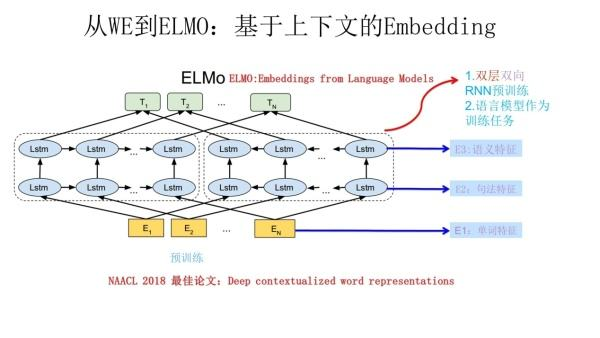它使用的是一个双向的 LSTM 语言模型，目标函数就是取这两个方向的语言模型的最大似然。

前向 LSTM：
p ( t 1 , t 2 , . . . , t N ) = ∏ k = 1 N p ( t k ∣ t 1 , t 2 , . . . , t k − 1 ) p(t_1,t_2,...,t_N)=\prod^N_{k=1}p(t_k|t_1,t_2,...,t_{k-1})
反向 LSTM：
p ( t 1 , t 2 , . . . , t N ) = ∏ k = 1 N p ( t k ∣ t k + 1 , t k + 2 , . . . , t N ) p(t_1,t_2,...,t_N)=\prod^N_{k=1}p(t_k|t_{k+1},t_{k+2},...,t_N)
最大似然函数：
∑ k = 1 N ( l o g p ( t k ∣ t 1 , t 2 , . . . , t k − 1 ) + l o g p ( t k ∣ t k + 1 , t k + 2 , . . . , t N ) ) \sum^N_{k=1}(logp(t_k|t_1,t_2,...,t_{k-1})+logp(t_k|t_{k+1},t_{k+2},...,t_N))
其中 ( t 1 , t 2 , . . . , t N ) (t_1,t_2,...,t_N) 是一系列的 tokens，对每一个 tokens，一个 L 层的双向 LSTM 要计算出 L+1 个表征（词向量），我们可以取最后的一个表征作为我们需要的词向量，也可以综合所有的表征做加权求和得到最终结果。

2.1 具体步骤

对于一个 supervise NLP 任务，可以分为三步：

• 产生预训练好的双向语言模型，模型由两层的双向 LSTM 组成，之间可由残差连接
• 在任务语料上 finetuning（无监督训练）进一步得到语言模型
• 利用 ELMo 的 word embedding 进行上层任务的训练

3. 模型评价

3.1 优点

1. ELMo 训练词向量是基于上下文变化而改变的，所以在一词多意方面 ELMo 的效果一定比 word2vec 要好。

2. ELMo 利用了双向的 LSTM 模型，能看到更长的上下文信息，更加准确代表一个词的意思。

3. ELMo 还有一个优势，就是它建立语言模型的时候，可以运用非任务的超大语料库去学习，一旦学习好了，可以平行的运用到相似问题上。

3.2 缺点

1. ELMo 对双向 LSTM 模型的输出只是采取的简单的拼接，并不能很好地融合双向的语义信息。
2. 双向 LSTM 模型对语义的提取不如 Transformer。

六、GPT

1. 简单介绍

GPT 是一种半监督的处理语言理解任务的模型，使用非监督的预训练和监督方式的微调。模型的目标是学习一个通用的表示，经过很小的调整就能在大量任务上进行应用，而且这个模型不需要目标任务和非标注的数据集在同一个领域，模型分为两个阶段：

1. 用语言模型预训练好一个深度模型
2. 使用相应的有标签的数据将这个模型的参数调整到目标任务

2. 模型结构和基本原理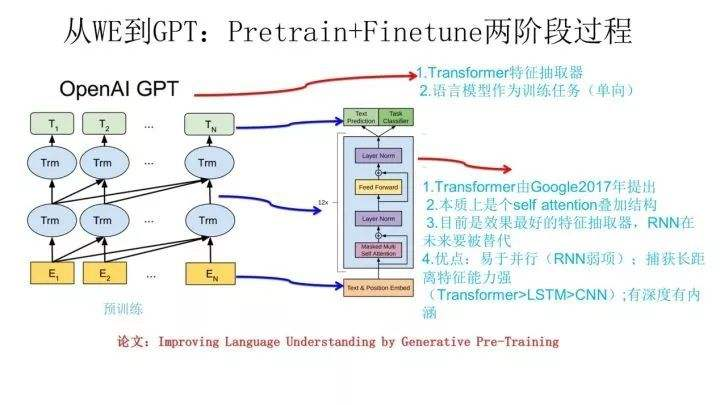2.1 无监督预训练

预训练过程是对非监督文本 ( x 1 , x 2 , . . . , x m